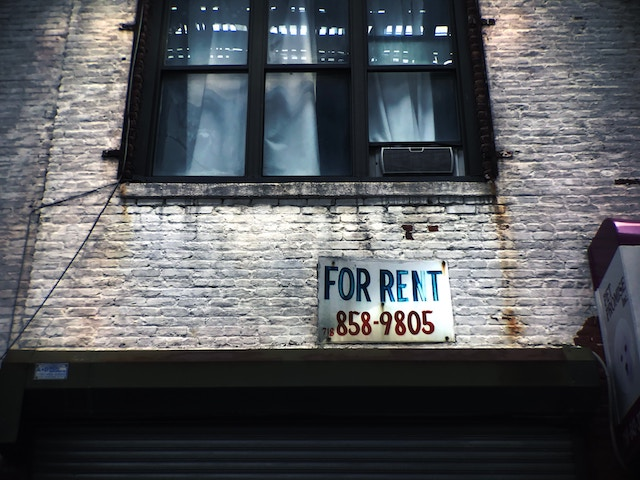Select PageOne of the most challenging aspects of finding an investment property is determining whether or not that property will make a good investment. However, there are a few methods investors can use for calculating the value of a potential investment property.

### The Rule of One Percent

This one percent rule is a way for investors to find properties that work for them. According to this rule, a property must rent for at least one percent of the total upfront cost.

With this rule in place, an investor should rent a \$100,000 property for \$1,000 a month. This helps to keep investors from losing money on rentals. However, it is important to remember that this rule applies to the total upfront cost of the property. This means that investors must combine the purchase price, any renovation costs, closing costs, and other expenses required to make the property rentable.

A property that passes this rule would make a good investment. If it doesn’t, it isn’t worth sinking money into.

### The Cap Rate

After narrowing down property options, investors need to research the capitalization rate. This “cap rate” helps to calculate the property’s ROI. This cap rate can be calculated by dividing a property’s total operating expenses by the purchase price. Find this rate using the following methods:

• The gross income is found by taking an average of the monthly rent for the property by multiplying the number by 11.5. This will calculate the amount that an investor can earn with the property, allowing that there is a vacancy for two weeks per year.
• Subtract the monthly operating expense from the gross income. This will total as the net income.
• Divide the net income of the property by the price of purchase to find the cap rate.
• Then, multiply this rate by 100. This will calculate the percentage of potential returns for the property.

When calculating the monthly operating expenses, be sure to avoid including the mortgage payment, as this shouldn’t be included in the cap rate. The calculation of the cap rate works off of the assumption that the property has been paid for in cash.

When it comes to ROI, each investor must do their own research and calculations to find the proper cap rate. Investors should keep in mind that the cap rate should be high enough to make the investment worth it.